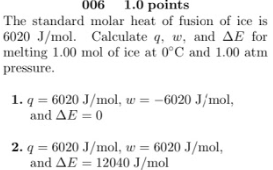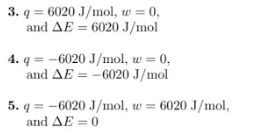# The standard molar heat of fusion of ice is 6020 J/mol. Calculate q, w, and ΔE for melting 1.00 mol ice at 0°C and 1.00 atm pressure. 1. q = 6020 J/mol, w = -6020 J/mol, and ΔE = 0 2. q = 6020 J/mol, w = 6020 J/mol, and ΔE = 12040 J/mol 3. q = 6020 J/mol, w = 0, and ΔE = 6020 J/mol 4. q = -6020 J/mol, w = 0, and ΔE = -6020 J/mol 5. q = -6020 J/mol, w = 6020 J/mol, and ΔE = 0